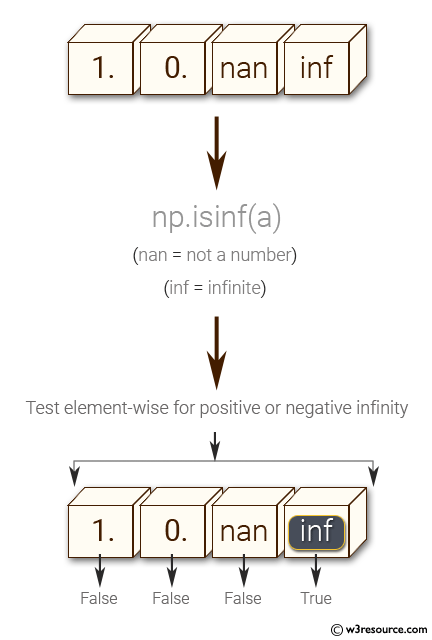﻿ NumPy: Test element-wise for positive or negative infinity - w3resource

# NumPy: Test element-wise for positive or negative infinity.

## NumPy: Basic Exercise-6 with Solution

Write a NumPy program to test element-wise for positive or negative infinity.

Sample Solution :

Python Code :

``````import numpy as np
a = np.array([1, 0, np.nan, np.inf])
print("Original array")
print(a)
print("Test element-wise for positive or negative infinity:")
print(np.isinf(a))
``````

Sample Output:

```Original array
[  1.   0.  nan  inf]
Test element-wise for positive or negative infinity:
[False False False  True]
```

Pictorial Presentation:Python Code Editor:

Have another way to solve this solution? Contribute your code (and comments) through Disqus.

What is the difficulty level of this exercise?

﻿

Inviting useful, relevant, well-written and unique guest posts